# Hands-on ActivityThe Balancing Act

(0 Ratings)

### Quick Look

Grade Level: 6 (6-8)

Time Required: 45 minutes

The time required does not include build/setup time.

Expendable Cost/Group: US \$0.00

This activity requires use of non-expendable (reusable) LEGO MINDSTORMS EV3 robotics kit, LEGO Fun with Bricks set and a set of gram masses; see the Materials List for details.

Group Size: 3

Activity Dependency: None

Subject Areas: Algebra, Number and Operations

### Summary

Students visualize and interact with concepts already learned, specifically algebraic equations and solving for unknown variables. They construct a balancing seesaw system (LEGO® Balance Scale) made from LEGO MINDSTORMS® parts and digital components to mimic a balancing scale. They are given example algebraic equation problems to analyze, configure onto the balance scale, and evaluate by manipulating LEGO pieces and gram masses that represent terms of an equation such as unknown variables, coefficients and integers. Digital color sensors, built into the LEGO Balance Scale, detect any balance or imbalances displayed on the balancing scale. The LEGO Balance Scale interactively issues a digital indication of balance or imbalance within the system. If unbalanced, students continue using the LEGO Balance Scale until they are confident in their understanding of solving algebraic equations. The goal is for students to become confident in solving algebraic equations by fundamentally understanding the basics of algebra and real-world algebraic applications.

### Engineering Connection

All engineers use equations to express different types of equilibriums that are seen throughout all types of systems used in our everyday lives. For instance, civil engineers must understand equilibrium forces in order to design bridges and buildings that do not collapse, where the supporting force is equivalent to the load force being applied. All engineers must understand equilibrium in order to keep balanced budgets of the funds available for projects that are equivalent to or greater than the funds being spent in order for the project to be successful. Electrical engineers must understand equilibrium in terms of the law of electricity, in which an electrical circuit is at equilibrium if the voltage produced in the circuit is equivalent to the total resistance times the current traveling through the circuit's electrical components. With electricity at equilibrium, engineers can build electrical circuits and devices such as computers, mobile phones and electric cars. Mechanical engineers must understand equilibrium for mechanical sustainability in order to place rovers on Mars or a person on a moon. All of these engineering equilibrium concepts require balanced equations; otherwise detrimental consequences can occur. For example, buildings and bridges would collapse, businesses and big projects would go bankrupt, electrical devices would be faulty or cause fires, and the \$2.6 billion NASA rovers would crash land onto the surface of Mars. Thus, the concept of equilibrium and balancing equations is a vital and important skill across all engineering disciplines.

In addition, this activity maintains a technological connection to engineering through the use of color sensors. Designed and used by engineers, color sensors are one of the many digital components that modern robots use for sensory and autonomous navigation. Color sensors can detect the difference between light and dark contrast, which can be instrumental in visual sensory for navigation through a particular pathway, determining light vs. dark, for example night vs. day or shadow vs. no shadow, or perhaps picking up various objects of varying light and dark contrasts.

### Learning Objectives

After this activity, students should be able to:

• Explain the principle idea of a "balanced" equation.
• Balance and solve one- or two-step algebraic equations.
• Describe the purpose and usage of a LEGO MINDSTORMS EV3 Intelligent Brick and sensors (especially a color sensor).

### Educational Standards Each TeachEngineering lesson or activity is correlated to one or more K-12 science, technology, engineering or math (STEM) educational standards. All 100,000+ K-12 STEM standards covered in TeachEngineering are collected, maintained and packaged by the Achievement Standards Network (ASN), a project of D2L (www.achievementstandards.org). In the ASN, standards are hierarchically structured: first by source; e.g., by state; within source by type; e.g., science or mathematics; within type by subtype, then by grade, etc.

###### Common Core State Standards - Math
• Understand solving an equation or inequality as a process of answering a question: which values from a specified set, if any, make the equation or inequality true? Use substitution to determine whether a given number in a specified set makes an equation or inequality true. (Grade 6) More Details

Do you agree with this alignment?

• Use variables to represent numbers and write expressions when solving a real-world or mathematical problem; understand that a variable can represent an unknown number, or, depending on the purpose at hand, any number in a specified set. (Grade 6) More Details

Do you agree with this alignment?

• Understand that positive and negative numbers are used together to describe quantities having opposite directions or values (e.g., temperature above/below zero, elevation above/below sea level, credits/debits, positive/negative electric charge); use positive and negative numbers to represent quantities in real-world contexts, explaining the meaning of 0 in each situation. (Grade 6) More Details

Do you agree with this alignment?

• Write and evaluate numerical expressions involving whole-number exponents. (Grade 6) More Details

Do you agree with this alignment?

• Solve real-world and mathematical problems by writing and solving equations of the form x + p = q and px = q for cases in which p, q and x are all nonnegative rational numbers. (Grade 6) More Details

Do you agree with this alignment?

• Use variables to represent quantities in a real-world or mathematical problem, and construct simple equations and inequalities to solve problems by reasoning about the quantities. (Grade 7) More Details

Do you agree with this alignment?

• Solve linear equations in one variable. (Grade 8) More Details

Do you agree with this alignment?

###### International Technology and Engineering Educators Association - Technology
• Students will develop an understanding of the relationships among technologies and the connections between technology and other fields of study. (Grades K - 12) More Details

Do you agree with this alignment?

• Explain how knowledge gained from other content areas affects the development of technological products and systems. (Grades 6 - 8) More Details

Do you agree with this alignment?

• Compare and contrast the contributions of science, engineering, mathematics, and technology in the development of technological systems. (Grades 6 - 8) More Details

Do you agree with this alignment?

###### New York - Math
• Understand solving an equation or inequality as a process of answering a question: which values from a specified set, if any, make the equation or inequality true? Use substitution to determine whether a given number in a specified set makes an equation or inequality true. (Grade 6) More Details

Do you agree with this alignment?

• Use variables to represent numbers and write expressions when solving a real-world or mathematical problem; understand that a variable can represent an unknown number, or, depending on the purpose at hand, any number in a specified set. (Grade 6) More Details

Do you agree with this alignment?

• Understand that positive and negative numbers are used together to describe quantities having opposite directions or values (e.g., temperature above/below zero, elevation above/below sea level, credits/debits, positive/negative electric charge); use positive and negative numbers to represent quantities in real-world contexts, explaining the meaning of 0 in each situation. (Grade 6) More Details

Do you agree with this alignment?

• Write and evaluate numerical expressions involving whole-number exponents. (Grade 6) More Details

Do you agree with this alignment?

• Solve real-world and mathematical problems by writing and solving equations of the form x + p = q and px = q for cases in which p, q and x are all nonnegative rational numbers. (Grade 6) More Details

Do you agree with this alignment?

• Use variables to represent quantities in a real-world or mathematical problem, and construct simple equations and inequalities to solve problems by reasoning about the quantities. (Grade 7) More Details

Do you agree with this alignment?

• Solve linear equations in one variable. (Grade 8) More Details

Do you agree with this alignment?

Suggest an alignment not listed above

### Subscribe

Get the inside scoop on all things TeachEngineering such as new site features, curriculum updates, video releases, and more by signing up for our newsletter!
PS: We do not share personal information or emails with anyone.

### Materials List

Each group needs:

To share with the class:

Alternative: LEGO MINDSTORMS NXT Set:

Note: This activity can also be conducted with the older (and no longer sold) LEGO MINDSTORMS NXT set instead of EV3; see below for those supplies:

• LEGO MINDSTORMS NXT robot, such as NXT Base Set
• computer loaded with the NXT 2.1 software

### More Curriculum Like This

High School LessonDoing the Math: Analysis of Forces in a Truss Bridge

Learn the basics of the analysis of forces engineers perform at the truss joints to calculate the strength of a truss bridge known as the “method of joints.” Find the tensions and compressions to solve systems of linear equations where the size depends on the number of elements and nodes in the trus...

### Pre-Req Knowledge

• A familiarity with the use of addition, subtraction, division and multiplication manipulation of integers within algebraic expressions.
• A beginner's understanding of one-variable algebraic equations.

### Introduction/Motivation

Entering into the world of the unknown can be a daunting experience; however it's not an avoidable experience! You have all been introduced to the world of the unknown when you were first introduced to the world of algebra. That's where we use letters and symbols to represent unknown quantities and numbers in mathematical statements known as equations. Understanding how to use and solve for these algebraic equations is a vital skill for everyone at any level of occupation, and not just engineers and scientists. However, in the beginning, algebra can be discouraging from frustration that sometimes occurs with learning and practicing algebra and complex math.

An equation is any mathematical statement that expresses two quantities to be equal indicated by an equal sign "=". For instance, the number of apples = the number of oranges is an example of a basic equation. Or perhaps, Temperature = yesterday's temperature + 5 °C. You might recognize Einstein's most famous equation, E = mc2, known as mass-energy equivalence formula. The phenomenon of this equation is beyond the scope of our activity, but it brings home the point that even Einstein utilized a basic form of an equation!

Equations are typically seen in the form similar to 3x + 2 = 5, where "x" indicates an unknown value or quantity known as the variable, "3" is the coefficient of x, "2" and "5" are constants that are represented as known quantitative values, and "+" is a mathematical operator. The "=" sign states items on the right and left side of the = sign are equivalent. One key note, if a quantity is not a constant, then it is a variable.

In this activity, we will use an interactive method in which you will be able to practice what you've learned about solving algebraic equations through the literal sense of what it means to be an equation: to be equal, to be balanced. The main goal for solving algebraic equations is to get the variable on one side of the equal sign by itself (that is,. x = some number). To eliminate a term on one side of the equation, you must use the opposite mathematical operation known as the inverse operation. This technique is typically taught as: Whatever you do to one side of the equation, you must do to the other side in order to keep the equation balanced. The easiest way to solidify this concept is to think of a balancing scale.

If you were to add weight to one side of the balance scale, you must add weight to other side of the balance scale in order to keep it balanced and leveled!

If you were to only add weight to only one side of the balance scale, the balance scale will lean to one side and it becomes imbalance, as shown below!

The same thing goes for equations. If you change one side of the equation, you must make the same equal change to the other side of the equation in order for the equation to remain balanced and equal! Here's an example of solving the equation x + 2 = 5, shown in Figures 1 - 5. You must first subtract 2 from the right side of the equal sign and then do exactly the same operation to the left side of the equal sign. The end results you'll get is x = 3.

Always check your answer by substituting the value back into the original equation and verifying that the quantities on both side of the equation are equaled, and thus, balanced!

The above example is an example of a one-step equation. A one-step equation is literally an equation that takes one step to solve. A two-step equation is literally an equation that takes two steps to solve. In the activity today, we will be practicing both types of these equations and checking your answers using a balance scale built using the LEGO MINDSTORMS EV3 parts, LEGO pieces and mass weights. We will represent unknown variables using a LEGO EV3 Intelligent Brick and constants using multiples of 1, 5, 10 or 20-gram masses. For instance, a constant valued as 5 will be represented using a 5 gram mass.

### Procedure

Background

PARTS TO KNOW:

The pieces that are used on the LEGO Balance Scale are shown in Figure 6. The gram masses can be labeled with a marker or paint to show which ones represent positive constants and which ones represent negative constants.

The LEGO Balance Scale is constructed before the activity begins. Figure 7 shows illustrations of the balance scale with and without a Balance Indicator. The color sensor is built into the balance scale's tower and hidden behind the Balance Indicator. The Balance Indicator's black and yellow LEGO blocks are used in order for the color sensor to detect dark (black blocks) and light (yellow blocks) variations when the scale is balanced or imbalanced when the scale sways up and down to reach equilibrium. The color sensor will see yellow when the scale is balanced and leveled horizontally, otherwise the color sensor will see black when the scale is imbalanced and unleveled horizontally.

The necessary components from the LEGO MINDSTORMS EV3 includes programmable EV3 intelligent brick; 3 interactive servo motors; sound, color, ultrasonic and 2 touch sensors; and many other components and instructions. The LEGO Programmable EV3 Brick is used for programming the color sensor interaction with the LEGO Balance Scale. The color sensor is used to determine balance and imbalance detected on the scale, and the basket container is used to store masses and LEGO variable and constant pieces (see Figure 8) .

HOW IT WORKS:

Using a new example, 3x - 2 = 4, Figures 9 - 17 explain how to use the LEGO Balance Scale to solve for the unknown variable x.

Configure the equation onto the LEGO Balance Scale, shown in Figure 9. Note that since we have not solved for x and do not know its true value, the LEGO Balance Scale is initially unbalanced.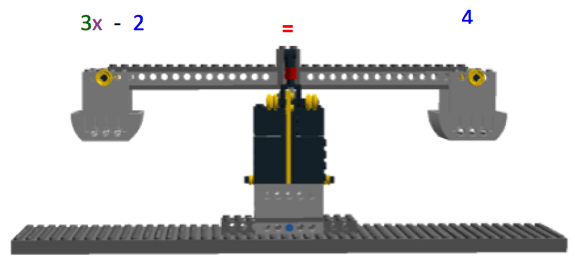Figure 9: Solving 3x – 2 = 4 with the LEGO Balance Scale.copyrightCopyright © 2013 Gisselle Cunningham, AMPS GK-12 Program, NYU-Poly

Figure 10 shows the LEGO pieces and the gram masses that represent the different terms in the equation 3x – 2 = 4.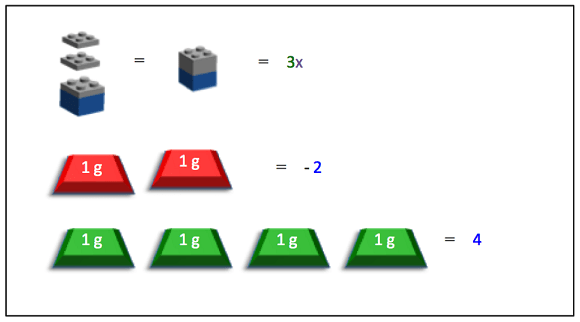Figure 10: LEGO pieces and gram masses used to represent all of the equation's components.copyrightCopyright © 2013 Gisselle Cunningham, AMPS GK-12 Program, NYU-Poly

Once all the LEGO pieces and gram masses for representing the equation components have been determined and gathered, they should be assembled on the LEGO Balance Scale, as shown in Figure 11.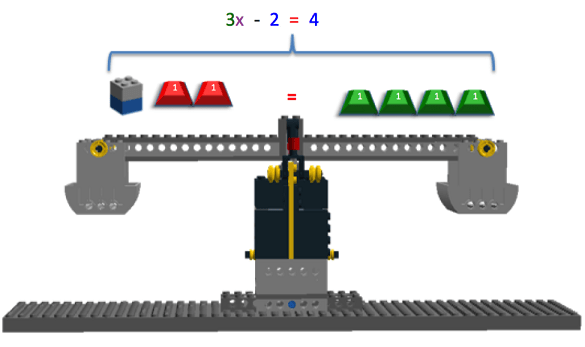Figure 11: LEGO pieces and gram masses in place on the LEGO Balance Scale.copyrightCopyright © 2013 Gisselle Cunningham, AMPS GK-12 Program, NYU-Poly

The next step is to represent the inverse operation needed to cancel out the "-2," which is to add a constant value of 2 to both sides of the equation. Therefore, two positive 1g masses are added to each side of the equation. On the left side, this cancels out the "-2." so both the two negative 1g masses and the two positive 1g masses are removed from the left side of the scale. This process is illustrated in Figures 12 and 13.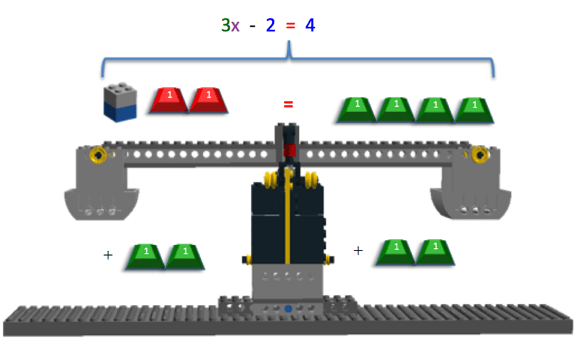Figure 12: Adding 2 to both sides cancels out the -2 on the left side of the equation.copyrightCopyright © 2013 Gisselle Cunningham, AMPS GK-12 Program, NYU-Poly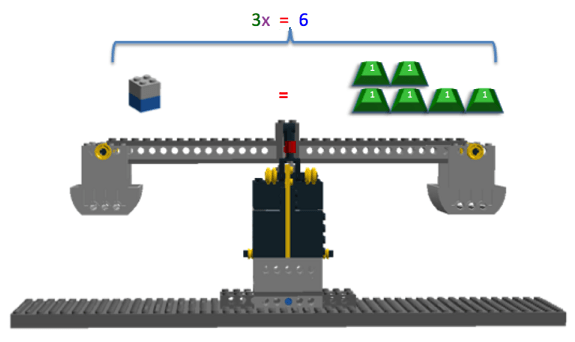Figure 13: The +2 and -2 cancelled to give a new equation 3x = 6.copyrightCopyright © 2013 Gisselle Cunningham, AMPS GK-12 Program, NYU-Poly

One last step must be performed to solve for x. Since x is multiplied by 3 in the equation 3x = 6, both sides of the equation need to be divided by 3, which is the inverse operation of multiplied by 3. On the left side, multiplying and dividing by 3 cancel out, leaving only x represented by the LEGO brick. On the right side of the equation, 6 is divided by 3, leaving the value of 2 represented by two positive 1g masses. This step is shown in Figure 14.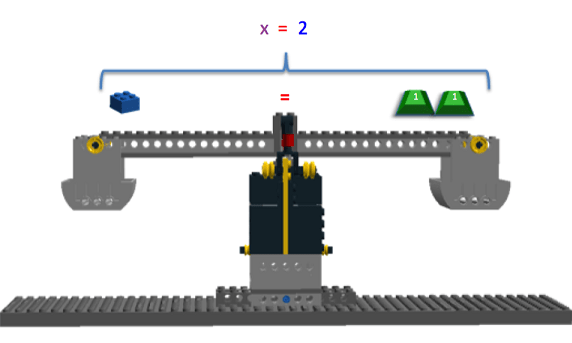Figure 14: The solution is x = 2!copyrightCopyright © 2013 Gisselle Cunningham, AMPS GK-12 Program, NYU-Poly

We have found a solution: x = 2! Once a solution is found, it must be checked to make sure it is correct. To check the answer, use the original equation, 3x – 2 = 4, and plug in the value of 2 for x. Figure 15 shows x replaced with the value of 2.

Before the Activity

1. Assemble one LEGO Balance Scale per group using available LEGO parts, LEGO EV3 MINDSTORMS brick and one color sensor. The LEGO instructional building guide for the LEGO Balance Scale is available at https://www.teachengineering.org/lego/building_instructions_balancing/building_instructions_balancing.html. The last page of the instructional building guide provides a full list of all the LEGO pieces used for building the LEGO Balance Scale. If necessary, it is okay to make part substitutions for LEGO bricks and pieces that you might not have. However, be aware of vital parts that are imperative to the make the LEGO Balance's seesaw mechanism work (such as the part labeled as the technic brick).
2. Connect a cable to the color sensor and port 1 of the LEGO EV3 Brick.
3. Program the LEGO EV3 Brick using the provided Balancing Act Program.
• You must install the LEGO MINDSTORMS EV3 software FIRST in order to program the brick with the Balance Act Program.
• Instructions (under Help -> Help and Support menu) are available in the LEGO EV3 MINDSTORMS software to assist you with getting started with EV3 programming, downloading the program to the brick and running the program.
• Other extremely useful tips and troubleshooting support is given as well (for example, information about cables, port and EV3 buttons). Be sure to take a look!
1. If necessary, review concepts of algebra, solving for unknown variables and the idea of balancing equations prior to starting the activity with students.
2. Before the start of the activity, give students the Balancing Equations Pre-Assessment.
3. One-step and two-step equations are provided in the Balancing Equations Activity Worksheet as examples to be completed during the activity; however additional examples can be made up. Keep in mind that examples and answers must be feasible using the masses that are available in the mass kit being used.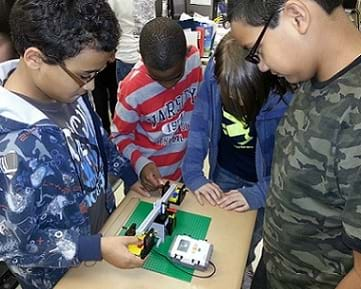Students interacting with the LEGO Balance Scale.copyrightCopyright © 2013 Gisselle Cunningham, AMPS GK-12 Program, NYU-Poly

With the Students

This activity can be separated into two methods of interaction with the LEGO Balance Scale.

• Option 1: First on paper and by hand, students are given an equation from the Balancing Equations Activity Worksheet to solve for the unknown variable. To verify their solution for the unknown variable, students use the LEGO Balance Scale.
• Option 2: Students are given an equation to initially configure on the LEGO Balance Scale. Students use the LEGO Balance Scale to solve for the unknown variable by moving pieces from the left and right sides of the balance scale. Students' solution is correct if their result is balanced.

Option 1 – Use the LEGO Balance Scale to verify the solution:

1. Turn on the LEGO MINDSTORMS EV3 Intelligent Brick by pressing the orange rectangular button.
2. Select the Balancing Act Program to run. Run the program
• A :-) icon appears on the LEGO MINDSTORMS EV3 Intelligent Brick screen signifying the LEGO Balance Scale is balanced and ready to use.
• If a :-( icon initially appears, then the LEGO Balance Scale must be calibrated to balance. Try adjusting the Balance Indicator in front of the color sensor to be sure that the yellow flat LEGO piece is directly in front of the color sensor. Additionally if necessary, use small LEGO pieces to calibrate the leveling of the LEGO Balance Scale by adding pieces to either the right or left side of the scale. Once balanced, a :-) icon appears and the LEGO Balance Scale is balanced and ready to use.
1. Give students an equation to solve for the unknown variable by hand and on paper.
2. To verify their result for the unknown variable, allow students to configure the original given equation on the LEGO Balance Scale using designated pieces to represent terms in the original equation (a LEGO brick for the unknown variable, LEGO plates for the unknown variable's coefficient, and green or red gram masses for positive or negative constants).
• Note: The LEGO Balance scale initially will be unbalanced until the unknown variable's LEGO brick is replaced with a corresponding gram mass.
• An example of how to configure the LEGO Balance Scale with designated pieces is provided in the Background section.
1. Students should substitute the LEGO brick that is configured on the LEGO Balance Scale representing an unknown variable with the solved value using a corresponding number of gram masses.
• Example: If the solved variable x = 5, then replace the LEGO brick with a 5 gram mass.
1. After the unknown variable has been substituted for its corresponding mass on the LEGO Balance Scale, the EV3 Intelligent Brick determines whether or not the result is correct and balanced.
• The LEGO Balance Scale initially begins to waver up and down to stabilize weight added to the scale.
• Once the LEGO Balance Scale is stabilized, the brick displays a :-) icon on screen if the LEGO Balance Scale is correctly balanced. Otherwise the brick displays a :-( icon on screen if the LEGO Balance scaled is not correctly balanced.
1. If the result is correct, congratulate students on a job well done! If a result is incorrect, encourage students to go back and try the problem again until their results end with a :-).

Option 2 – Use LEGO Balance Scale to Solve and Verify Solution:

1. Turn on the brick by pressing the orange rectangular button.
2. Select the Balancing Act Program to run. Run the program.
• A :-) icon appears on the brick screen signifying the LEGO Balance Scale is balanced and ready to use.
• If a :-( icon initially appears, then the LEGO Balance Scale must be calibrated to balance. Try adjusting the Balance Indicator in front of the color sensor to be sure that the yellow flat LEGO piece is directly in front of the color sensor. Additionally if necessary, use small LEGO pieces to calibrate the leveling of the LEGO Balance Scale by adding pieces to either the right or left side of the scale. Once balanced, a :-) icon appears and the LEGO Balance Scale is balanced and ready to use
1. Give students an equation to solve for the unknown variable using the LEGO Balance Scale.
2. Allow students to configure the given equation on the LEGO Balance Scale using a LEGO brick for the unknown variable, LEGO plates for the unknown variable's coefficient, and green or red gram masses for positive or negative constants, respectively.
• Note: The LEGO Balance scale will initially be unbalanced until the unknown variable's LEGO brick is replaced with a corresponding gram mass.
• An example of how to configure the LEGO Balance Scale with designated pieces is provided in the Background section.
1. Using the LEGO Balance Scale, allow students to directly solve for the unknown variable using algebraic and balancing equation manipulation techniques that they have already learned in class. Allow students to note their solutions on paper for the unknown variable.
• An example of how to solve equations using the LEGO Balance Scale is provided in the Background section.
1. To verify a value for the unknown variable, allow students to reconfigure the original equation on the LEGO Balance Scale using a LEGO brick for the unknown variable, LEGO plates for the unknown variable's coefficient, and green or red gram masses for positive or negative constants, respectively.
2. Students should substitute the brick with corresponding gram masses equal to the solved value they've found.
• Example: If the solved variable x = 3, then replace the brick with three 1 gram mass.
1. After the unknown variable has been substituted for its corresponding mass on the LEGO Balance Scale, the brick determines whether or not the result is correct and balanced.
• The LEGO Balance Scale initially begins to waver up and down to stabilize weight added to the scale.
• Once the LEGO Balance Scale is stabilized, the brick displays a :-) icon on screen if the LEGO Balance Scale is correctly balanced. Otherwise the brick displays a :-( icon on screen if the LEGO Balance scaled is not correctly balanced.
1. If the result is correct, congratulate students on a job well done! If a result is incorrect, encourage students to go back and try the problem again until their results end with a :-).

### Vocabulary/Definitions

balanced equation: An equation that has equal values on both side of the equal sign.

coefficient: A constant numerical quantity placed before (multiplied by) the unknown variable in an algebraic equation or expression.

color sensor: A sensor that can distinguish between different shadings of light and dark. A color sensor is typically used on robots to help them "see" and detect objects of different light and dark contrast.

constant: A value that does not change.

equation: A mathematical statement that shows that two quantities are equal.

inverse operation: A mathematical operation that is opposite of the mathematical operation being observed.

LEGO MINDSTORMS: A robotics kit made by the LEGO Corporation that contains software and hardware to create small, programmable and customizable robots. Kits include a programmable "brick" computer that controls the system, LEGO parts and a set of modular sensors and motors to create mechanical systems.

one-step equation: An equation that can be solved in one step.

two-step equation: An equation that can be solved in two steps.

variable: A letter or symbol representing an unknown value. This is the quantity that is being solved for in an algebraic equation.

### Assessment

Pre-Activity Assessment

The Balancing Equations Pre-Assessment provides generic questions regarding the main principles, usage and solving of equations in order to determine where students' mishaps may fall and where to focus the activity.

Activity Embedded Assessment

The Balancing Equations Activity Worksheet provides comprehensive questions during the completion of this activity to provoke students' critical thinking in a sequential manner.

Post-Activity Assessment

The Balancing Equations Post-Assessment provides comprehensive questions after the completion of this activity to determine students' improvements and opinion based on solving equations.

### Safety Issues

• This activity is not suitable for small children based on the small LEGO pieces, which can be a choking hazard.

### Troubleshooting Tips

Make sure the yellow brick of the Balance Indicator is configured directly in front of the color sensor in order for the color sensor to detect light to represent a balanced LEGO Balance Scale.

Make sure the LEGO Balance Scale is leveled and balanced prior to solving equations. It may be necessary to calibrate the scale's sides by adding small LEGO pieces to distribute the weight correctly.

Make sure equivalent parts are used on both sides of the LEGO Balance Scale to ensure balance.

The LEGO MINDSTORMS EV3 software has helpful tips and support on getting started with EV3 programming and more under its Help menu.

It can be tricky to solve problems with both positive and negative constants. We recommend differentiating positive constants and negative constants by assigning the gram masses (or the pennies) with a color. For example, a red-marked penny would represent a positive constant, while a blue-marked penny would represent a negative constant.

### Additional Multimedia Support

© 2013 by Regents of the University of Colorado; original © 2012 Polytechnic Institute of New York University

### Contributors

Gisselle Cunningham, Russell Holstein, Linderick Outerbridge, Jared Soto, Timothy Li

### Supporting Program

AMPS GK-12 Program, Polytechnic Institute of New York University

### Acknowledgements

This activity was developed under the Applying Mechatronics to Promote Science (AMPS) Program funded by National Science Foundation GK-12 grant no. 0741714. However, these contents do not necessarily represent the policies of the NSF and you should not assume endorsement by the federal government.